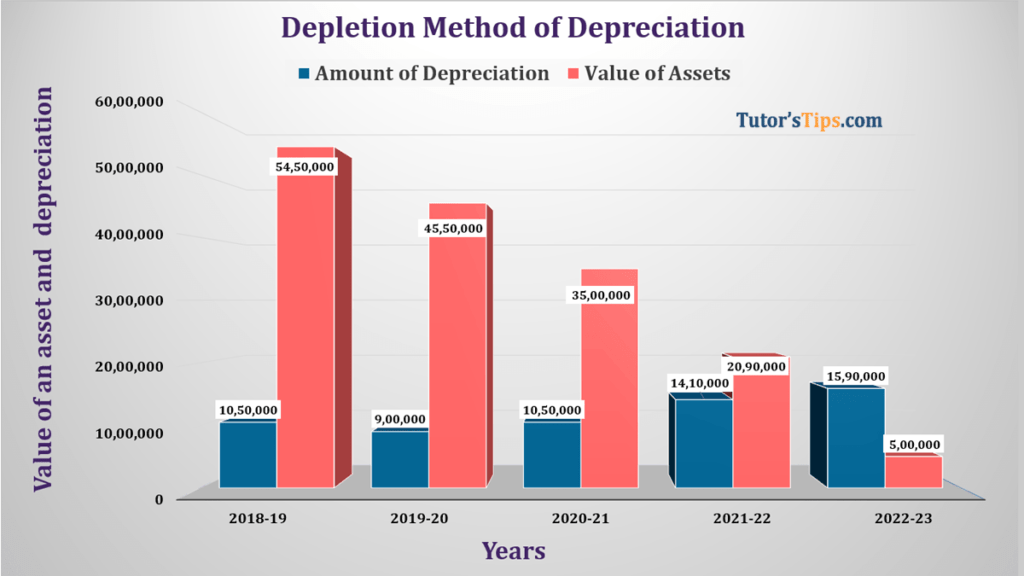# Depletion Method of Depreciation | ExampleDepletion Method of Depreciation is used for wasting assets like coal, mines, well and etc. The rate of dep is calculated by dividing the cost of an asset by the estimated quantity of product likely to be available. The amount of the dep can be calculated when we multiplying the total output of the financial year with the rate of depreciation.

### This method of depreciation is applied in the following cases: –

• Where we will use natural resources like the coal mine, oil reserves, etc.

### Example of Depletion Method of Depreciation:

The HPCL purchased land for extracting oil from it on 1st April 2018 at a cost of Rs 50,00,000/- and spent Rs. 10,00,000 on its development like construction of road and construction of drill pad. There will Drilling fee of Rs 4,00,000 and restoration cost of land is 1,00,000. The resale value of the land will be 5,00,000.

The company expect 2,00,000 barrels of oil from that land. The following table shows the total extraction from the land during the particular year in the next 5 years.

1. 31/03/2019 – 35,000
2. 31/03/2020 – 30,000
3. 31/03/2021 – 35,000
4. 31/03/2022 – 47,000
5. 31/03/2023 – 53,000

Calculate the amount of depreciation.

Solution : –

Calculation of the amount of depreciation under Depletion Method of Depreciation:-

Formula:-

So now, we will calculate the amount of depreciation with the above-shown formula:

Total Cost of an asset                                  =  Land Price + Development
Charges + Drilling Fee +
Restoration Cost

=  50,00,000 + 10,00,000+ 4,00,000
+1,00,000

=  65,00,000/-

Resale(Scrap) value of an asset              =  5,00,000/-

Total output from an asset(in barrels)=  2,00,000 barrels

So,

Depletion(Depreciation) – per barrels      =  65,00,000-5,00,000 / 2,00,000

=  Rs. 30 per barrels

So now, the following table shows the calculation of an amount of depreciation year by year:-

 Year Total output from an asset (in barrels) Rate of Depreciation (Per barrel) Amount of Depreciation for Year (Rs.) 2018-19 35,000 30 10,50,000 2019-20 30,000 30 9,00,000 2020-21 35,000 30 10,50,000 2021-22 47,000 30 14,10,000 2022-23 53,000 30 15,90,000

Journal entries or ledger are the same with the following methods: